×

Search anything:

# Applications of Autoencoders

#### Machine Learning (ML) autoencoderWelcome Friends,
Here, we are going to discuss the brief summary of Autoencoders and then come to it's practical applications.

## Main Idea behind Autoencoder is -

• Take data in some original(high-dimensional space);
• Then project data into a new space from which it can be accurately restored.

Autoencoders are neural networks that aim to copy their inputs to outputs.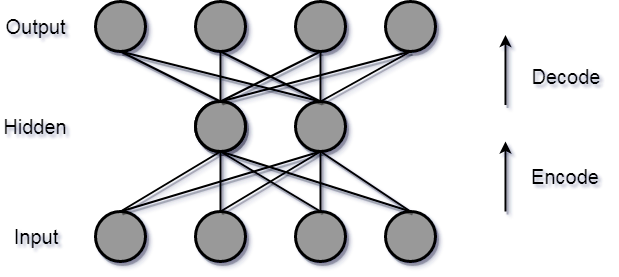Suppose data is represented as x.

• Encoder : - a function f that compresses the input into a latent-space representation. f(x) = h
• Decoder : - a function g that reconstruct the input from the latent space representation. g(h) ~ x.

Now, come to the main topic of our article, i.e.

## Applications of Autoencoders

1. Dimensionality Reduction
2. Image Compression
3. Image Denoising
4. Feature Extraction
5. Image generation
6. Sequence to sequence prediction
7. Recommendation system

Let's discuss each one of them -

# 1). Dimensionality Reduction using Undercomplete Autoencoders

Question - You might wonder, "How does feature learning or dimension reduction happen if the end result is same as input?".

Answer - You already have studied about the concept of Undercomplete Autoencoders, where the size of hidden layer is smaller than input layer. We force the network to learn important features by reducing the hidden layer size.
Also, a network with high capacity(deep and highly nonlinear ) may not be able to learn anything useful. Dimension reduction methods are based on the assumption that dimension of data is artificially inflated and its intrinsic dimension is much lower. As we increase the number of layers in an autoencoder, the size of the hidden layer will have to decrease. If the size of the hidden layer becomes smaller than the intrinsic dimension of the data then it will result in loss of information.

Now, we define our undercomplete autoencoder model -

``````    #defining input placeholder for autoencoder model
input_img = Input(shape=(784,))
# "enc_rep" is the encoded representation of the input
enc_rep = Dense(2000, activation='relu')(input_img)
enc_rep = Dense(500, activation='relu')(enc_rep)
enc_rep = Dense(500, activation='relu')(enc_rep)
enc_rep = Dense(10, activation='sigmoid')(enc_rep)

# "decoded" is the lossy reconstruction of the input from encoded representation
decoded = Dense(500, activation='relu')(enc_rep)
decoded = Dense(500, activation='relu')(decoded)
decoded = Dense(2000, activation='relu')(decoded)
decoded = Dense(784)(decoded)

# this model maps an input to its reconstruction
autoencoder = Model(input_img, decoded)
``````

Here is an example of Image reconstruction with dimensionality reduction on Fashion MNIST dataset -

## Original Images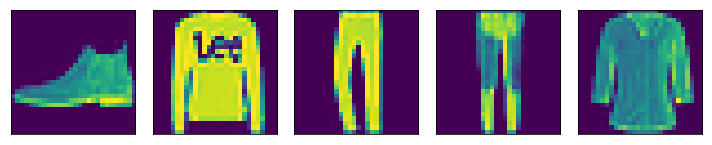## Images reconstructed from Autoencoder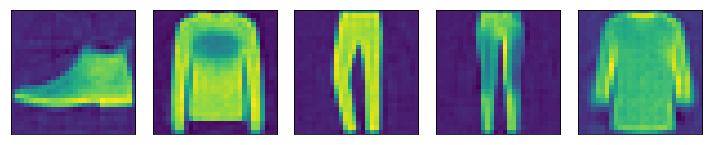Yeahh!! I am very happy with these results of our autoencoder.

Here, I am also providing you the link of my Google Colaboratory Python3 Notebook, where you can find the complete code for this autoencoder. Image_Reconstruction_from_Autoencoder.

Note :- Autoencoders are more powerful than PCA in dimensionality reduction.

# 2). Image Compression

Usually, Autoencoders are really not good for data compression.
For Image Compression, it is pretty difficult for an autoencoder to do better than basic algorithms, like JPEG and by being only specific for a particular type of images, we can prove this statement wrong. Thus, this data-specific property of autoencoders makes it impractical for compression of real-world data. One can only use them for data on which they were trained, and therefore, generalisation requires a lot of data.

# 3). Image Denoising

Today, Autoencoders are very good at denoising of images.
What happens when rain drops are on our window glass?
Ofcourse, we can't get a clear image of "What is behind the scene?". Here rain drops can be seen as noise. So,

When our image get corrupted or there is a bit of noise in it, we call this image as a noisy image.
To obtain proper information about the content of image, we want Image Denoising.
We define our autoencoder to remove (if not all)most of the noise of the image.

Let's take an example of MNIST Digit dataset.

1. We first add noise to our original data.

## Original Digits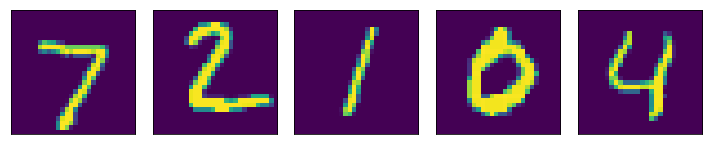## After adding noise to Digits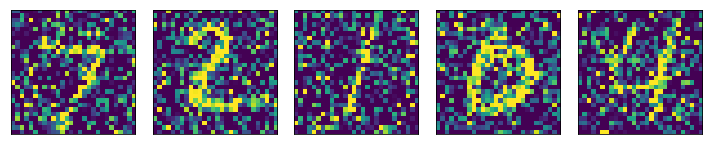2. We now define our denoising autoencoder as -

``````    Input_img = Input(shape=(28, 28, 1))

x1 = Conv2D(64, (3, 3), activation='relu', padding='same')(Input_img)
x1 = MaxPool2D( (2, 2), padding='same')(x1)
x2 = Conv2D(32, (3, 3), activation='relu', padding='same')(x1)
x2 = MaxPool2D( (2, 2), padding='same')(x2)
x3 = Conv2D(16, (3, 3), activation='relu', padding='same')(x2)
encoded    = MaxPool2D( (2, 2), padding='same')(x3)

# decoding architecture
x3 = Conv2D(16, (3, 3), activation='relu', padding='same')(encoded)
x3 = UpSampling2D((2, 2))(x3)
x2 = Conv2D(32, (3, 3), activation='relu', padding='same')(x3)
x2 = UpSampling2D((2, 2))(x2)
x1 = Conv2D(64, (3, 3), activation='relu')(x2)
x1 = UpSampling2D((2, 2))(x1)
decoded   = Conv2D(1, (3, 3), padding='same')(x1)

autoencoder = Model(Input_img, decoded)
``````

3. Denoised digits obtained from our autoencoder are -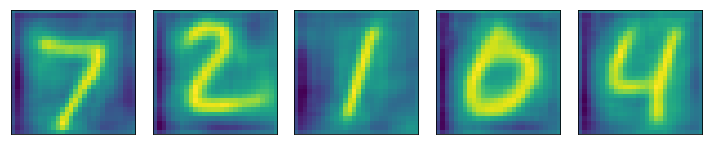Great Results!!! We can easily read our digits.
I am also sharing my Google Colaboratory Python3 Notebook for this complete code. Image Denoising Autoencoder.

# 4). Feature Extraction

Encoding part of Autoencoders helps to learn important hidden features present in the input data, in the process to reduce the reconstruction error. During encoding, a new set of combination of original features is generated.

# 5). Image Generation

There is a type of Autoencoder, named Variational Autoencoder(VAE), this type of autoencoders are Generative Model, used to generate images.

The idea is that given input images like images of face or scenery, the system will generate similar images. The use is to:

• generate new characters of animation
• generate fake human images

Example of Anime characters generated by variational autoencoder( Source - Image ) --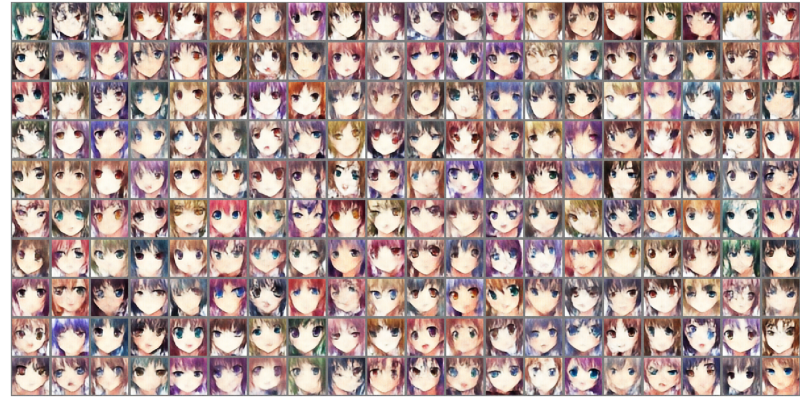A complete guide is provided by Francois Chollet on Variational Autoencoder.

# 6). Sequence to Sequence Prediction

The Encoder-Decoder Model that can capture temporal structure, such as LSTMs-based autoencoders, can be used to address Machine Translation problems.

This can be used to:

• predict the next frame of a video
• generate fake videos

A complete guide is provided by Jason Brownlee on Sequence to Sequence Prediction, where source sequence is a series of randomly generated integer values, such as [20, 36, 40, 10, 34, 28], and the target sequence is a reversed pre-defined subset of the input sequence, such as the first 3 elements in reverse order [40, 36, 20].
Other examples are -

``````    Source				Target
[13, 28, 18, 7, 9, 5]		[18, 28, 13]
[29, 44, 38, 15, 26, 22]		[38, 44, 29]
[27, 40, 31, 29, 32, 1]		[31, 40, 27]
``````

# 7). Recommendation Systems

Deep Autoencoders can be used to understand user preferences to recommend movies, books or other items. Consider the case of YouTube, the idea is:

• the input data is the clustering of similar users based on interests
• interests of users are denoted by videos watched, watch time for each, interactions (like commenting) with the video
• above data is captured by clustering content
• Encoder part will capture the interests of the user
• Decoder part will try to project the interests on twp parts:
• existing unseen content
• new content from content creators

# Stay Focussed and Keep Studying!!!#### Nidhi Mantri

Specialist Programmer at Infosys Ltd; Completed B. Tech in CS at IIIT Kota (2020); Machine Learning certification from deeplearning.ai; Project on Emotion Classification using CNN.

Applications of Autoencoders